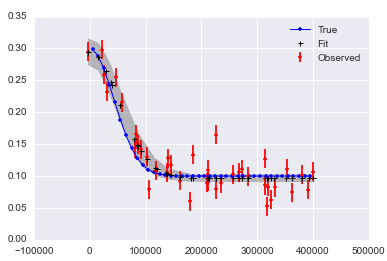# Category Archives: statistics

## Kish Stuff

A student came by interested in survey statistics and we go to talking about what an amazing person Leslie Kish must have been. We did some googling on it. Here are a few items we found:

Click to access Kish_Leslie_1977_edit_(wla_092809).pdf

Filed under statistics

## Non-parametric regression in Python: Gaussian Processes in sklearn (with a little PyMC)

I’ve got a fun class going this quarter, on “artificial intelligence for health metricians”, and the course content mixed with some of the student interest has got me looking at the options for doing Gaussian process regression in Python. `PyMC2` has some nice stuff, but the `sklearn` version fits with the rest of my course examples more naturally, so I’m using that instead.

But `sklearn` doesn’t have the fanciest of fancy covariance functions implemented, and at IHME we have been down the road of the Matern covariance function for over five years now. It’s in `PyMC`, so I took a crack at mash-up. (Took a mash at a mash-up?) There is some room for improvement, but it is a start. If you need to do non-parametric regression for something that is differentiable more than once, but less than infinity times, you could try starting here: http://nbviewer.ipython.org/gist/aflaxman/af7bdb56987c50f3812b

p.s. Chris Fonnesbeck has some great notes on doing stuff like this and much more here: http://nbviewer.ipython.org/github/fonnesbeck/Bios366/blob/master/notebooks/Section5_1-Gaussian-Processes.ipynb

Comments Off on Non-parametric regression in Python: Gaussian Processes in sklearn (with a little PyMC)

Filed under statistics

## Bayesian Correlation in PyMCIs there a nice, simple reference for just what exactly these graphical model figures mean? I want more of them.

Filed under statistics

## Statistics in Python: Calculating R^2

I wanted to include some old-fashioned statistics in a paper recently, and did some websearching on how to calculate R^2 in Python. It’s all very touchy, it seems. Here’s what I found:

I eventually went with this:

```%load_ext rmagic

x = np.array(1/df.J)
y = np.array(df.conc_rand)
%Rpush x y
%R print(summary(lm(y ~ x + 0)))
```

Comments Off on Statistics in Python: Calculating R^2

Filed under statistics

## CrossValidated on interesting and well-written papers in applied stats

I should read some of these, and stash a few for the PGF journal club:

http://stats.stackexchange.com/questions/9365/what-are-some-interesting-and-well-written-applied-statistics-papers

http://www.jstor.org/stable/2347679

Comments Off on CrossValidated on interesting and well-written papers in applied stats

Filed under statistics

## MCMC in Python: observed data for a sum of random variables in PyMC

I like answering PyMC questions on Stack Overflow, but sometimes I give an answer and end up the one with the question. Like what would you model as the sum of a Poisson and a Negative Binomial?

Comments Off on MCMC in Python: observed data for a sum of random variables in PyMC

Filed under statistics

## MCMC in Python: sim and fit with same model

Here is a github issue and solution that I saw the other day. I think it’s a nice pattern.

```def generate_model(values={'mu': true_param, 'm': None}):

#prior
mu = pymc.Uniform("mu", lower=-10, upper=10, value=values['mu'],
observed=(values['mu'] is not None))

# likelihood function
m = pymc.Normal("m", mu=mu, tau=tau, value=values['m'],
observed=(values['m'] is not None))

return locals()
```

Comments Off on MCMC in Python: sim and fit with same model

Filed under statistics

## MCMC in Python: Fit a non-linear function with PyMC

Here is a recent q&a on stack overflow that I did and liked.Comments Off on MCMC in Python: Fit a non-linear function with PyMC

Filed under statistics

## The one before that

Jake Vanderplas’s comparison of Python MCMC modules was preceded by a Bayesian polemic. In general, I find the stats philosophy war old-timey and distracting, but his comparison of confidence intervals and credible intervals is something I need to understand better.

http://jakevdp.github.io/blog/2014/06/12/frequentism-and-bayesianism-3-confidence-credibility/

Comments Off on The one before that

Filed under statistics

## MCMC in Python: a bake-off

While I’m on a microblogging spree, I’ve been meaning to link to this informative comparison of pymc, emcee, and pystan: http://jakevdp.github.io/blog/2014/06/14/frequentism-and-bayesianism-4-bayesian-in-python/

Comments Off on MCMC in Python: a bake-off

Filed under statistics